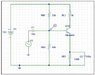# Help on BJT question

• darkwhite87

#### darkwhite87

Please view the attached circuit. I will be appreciated for any suggested answer, thanks!

Q1) Calculate the dc input resistance of the transistor amplifier shown in figure 1 below if the current gain of the transistor is 400:
I need some help on this one, i don't know the relationship between input resistance and current gain, isn't input resistance independent and also the same regardless of transistor current gain??

Q2) What is the purpose of Capacitors C1 and C2?
MY ANS: decoupling capacitor to keep the bias voltage V_BE constant

#### Attachments

•question.JPG
20.5 KB · Views: 682

Does current gain mean beta here?
I believe C1 is a DC blocking cap, in case your voltage source has a DC offset to it. Your using R1 and R2 to do all the biasing. I think assuming C2 is picked carefully, it will short out during the flat band. Draw the hybrid pi model and derive the voltage gain with C2 shorting to ground or removed from the circuit. What do you see.

C1 is called a coupling capacitor. It keeps the base bias voltage constant. C2 is used to "bypass" the resistor RE1 at the signal frequency.

Like Corneo said, draw the hybrid pi model to find the transistors base "resistance". I believe you could then find the circuits input resistance by adding this base "resistance" to the parallel combination of RB1 & BR2.

Thanks for the hint, this is wat i have done so far as implementing pi-model.

The input resistance should be 33k//68k//R_pi (pi-model circuit attached)

But how do i obtain the value for R_pi, is it possible to obtain it from the given data "current gain of transistor is 400"?? if not, why does the question give this value?

#### Attachments

" The input resistance should be 33k//68k//R_pi (pi-model circuit attached)"
Look at the pi model again. The emitter should not be grounded in this case and hence the 33k//69k combination should be in series with R_pi. To find R_pi you should look at your text to see if you can find how the base-emitter voltage and base current might be used.

dlgoff, I though C2 shorts out during midband and hence the emitter shorts to ground.

assuming beta = 400, i think rpi = beta/gm = beta/(Ic/Vt) = Vt/Ib

Last edited:
dlgoff, I though C2 shorts out during midband and hence the emitter shorts to ground.

assuming beta = 400, i think rpi = beta/gm = Ic/Vt

gm = Ic/Vt; and r_pi = beta/gm, so that

r_pi = beta / (Ic/Vt) = (beta*Vt)/Ic

In the part data sheets, beta, which is current gain, is labeled as "hFE" for dc and low frequency value, and "hfe" at high frequency.

Lets see. V_be = I_b x R_pi and since I_b = I_c / beta

R_pi = V_be / I_b =( 0.7 x beta)/I_c Note: for a npn silicon junction transistor, V_be is approx .7volts

Can we find the collector current I_c?

Lets see. V_be = I_b x R_pi and since I_b = I_c / beta

R_pi = V_be / I_b =( 0.7 x beta)/I_c Note: for a npn silicon junction transistor, V_be is approx .7volts

Can we find the collector current I_c?

I beg to differ. R_pi = vbe/ib, noting the lower case "v" in vbe, as opposed to capital V in Vbe. The dc or bias value is Vbe, approx. 0.65 or 0.7 volts, but vbe is the ac small signal swing, typically fractions of a millivolt. R_pi is the small signal ac swing "vbe" divided by ib, the small signal ac swing as well. R_pi also equals hfe/gm, noting that "hfe" is the ac current gain. This can be found in any college level text.

Yes you are right. The V & I should have been v & i for the small-signal model. Thanks

Your first simplification is wrong. You paralleled RB1 and RB2 together! Your trying to find the DC biasing condition first. Make sure your transistor is operating in the forward active region before you go about determining the voltage gain.

Hint: Voltage Divider.

For the first simplification I used the Thevinin's Theorem.

Looking at the circuit to left of base, the total resistance is paralleled RB1 and RB2 (To find thevinin's resistance ground the voltage supply i think), and then find the thevinin voltage. so..is it really wrong?

No. That isn't right because one end of RB1 is connected to 12V and the other end is connected to the base. RB2 is connected to gnd and base. How are these resistors in parallel?

Corneo. He is determining the equivalent circuit. Yes you would use the divider to determine the base bias voltage. Vb=V1(Rb1/Rb1+Rb2). Then apply that to the equivalent resistance which is Rb1||Rb2.

darkwhite87. Shouldn't Rb1||Rb2 be in series with Rpi?

When using small signal analysis, the bypass capacitor shorts out midband, so emitter is grounded, also said by corneo in #6